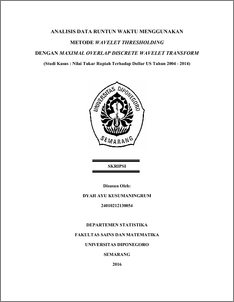# ANALISIS DATA RUNTUN WAKTU MENGGUNAKAN METODE WAVELET THRESHOLDING DENGAN MAXIMAL OVERLAP DISCRETE WAVELET TRANSFORM (Studi Kasus : Nilai Tukar Rupiah Terhadap Dollar US Tahun 2004 - 2014)

KUSUMANINGRUM, DYAH AYU (2017) ANALISIS DATA RUNTUN WAKTU MENGGUNAKAN METODE WAVELET THRESHOLDING DENGAN MAXIMAL OVERLAP DISCRETE WAVELET TRANSFORM (Studi Kasus : Nilai Tukar Rupiah Terhadap Dollar US Tahun 2004 - 2014). Undergraduate thesis, Fakultas Sains dan Matematika, Undip.Preview
PDF
1160Kb

## Abstract

Wavelet is a mathematical tool for analyzing time series data. Wavelet has certain properties one of which is localized in the time domain and frequency and form an orthogonal basis in the space L2(R). There are two types of wavelet estimators are linear and nonlinear wavelet estimators. Linear wavelet estimators can be analyzed using the approach of Multiresolution Analysis (MRA), while nonlinear wavelet estimator called Wavelet Thresholding. Wavelet thresholding are emphasizing the reconstruction of wavelet using a number of the largest coefficient or can be said that only coefficient greater than threshold value, while other coefficients are ignored. There’re Several factors affect the smoothness estimate on Wavelet Thesholding include the type of wavelet function, types of functions thresholding, thresholding parameters, and the level of resolution. On the issue of the problems is to have optimal threshold value. Based on the results of empirical studies of data of the rupiah against the US dollar using wavelet thresholding method produced a value Mean Square Error (MSE) that smaller compared to analysis using the method of approach Multiresolution Analysis (MRA). Analysis using Wavelet Thresholding with a case study of the rupiah against the US dollar is considered better than the analysis using MRA approach. Keywords: Multiresolution Analysis, Wavelet Estimator thresholding.

Item Type: Thesis (Undergraduate) H Social Sciences > HA Statistics Faculty of Science and Mathematics > Department of Statistics 55057 Mr Hasbi Yasin 26 Jul 2017 08:45 26 Jul 2017 08:45

Repository Staff Only: item control page